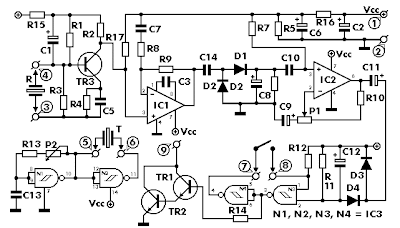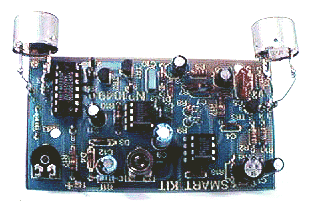Componets
R1 = 180 KOhm C1, 6 = 10uF/16V TR1, 2, 3 = BC547 , BC548
R2 = 12 KOhm C2 = 47uF/16V P1 = 10 KOhm trimmer
R3, 8 = 47 KOhm C3 = 4,7 pF P2 = 47 KOhm trimmer
R4 = 3,9 KOhm C4, 7 = 1 nF IC1, 2 = 741 OP-AMP
R5, 6, 16 = 10 KOhm C5 = 10nF IC3 = 4093 C-MOS
R7, 10, 12, 14, 17 = 100 K? C8, 11 = 4,7 uF/16V R = TRANSDUCER 40KHz
R9, 11 = 1 MOhm C9 = 22uF/16V T = TRANSDUCER 40KHz
R13, 15 = 3,3 KOhm C10 = 100 nF D1, 2, 3, 4 = 1N4148
C12 = 2,2 uF/16V
C13 = 3,3nF
C14 = 47nF

This is a very interesting project with many practical applications in security and alarm systems for homes, shops and cars. It consists of a set of ultrasonic receiver and transmitter which operate at the same frequency. When something moves in the area covered by the circuit the circuit’s fine balance is disturbed and the alarm is triggered. The circuit is very sensitive and can be adjusted to reset itself automatically or to stay triggered till it is reset manually after an alarm.

This site uses Akismet to reduce spam. Learn how your comment data is processed.